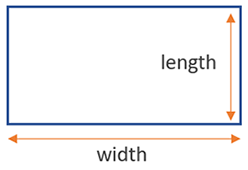# Perimeter of a Rectangle Calculator

Use this calculator to easily calculate the perimeter of a rectangle given its sides (length and width) in any metric: mm, cm, meters, km, inches, feet, yards, miles...

### Calculation results

14 cm Rectangle perimeter
Share calculator:

Embed this tool:
get code

## Perimeter of a rectangle formula

The formula for the perimeter of a rectangle is (width + height) x 2, as seen in the figure below:This is the equivalent of adding all four sides, since opposite sides are of equal length by definition. Make sure the calculation is done using the same unit - the result will also be in that unit, e.g. mm, cm, meters, km, or in, ft, yd, miles.

## How to calculate the perimeter of a rectangle?

First, measure each side and record the measurement using the same units. If you are working off a plan or schematic, you already have the measurement. Then, use the formula above, or use our perimeter of a rectangle calculator, whichever is easier for you. It's as simple as that.

The applications of rectangular geometry are key in various sciences and practical scenarios, e.g. in construction, engineering, home decoration, landscaping, and others.

## Example: find the perimeter of a rectangle

Say there is a rectangular garden and a wall needs to be raised on all sides of it. How long should the wall be? The answer is that it needs to be as long as the perimeter of the rectangular garden. If one side of the garden is 30 feet and the other is 10 feet, what is its perimeter? The answer is simply 30 x 10 = 300 feet. So one would need to build a 300-feet wall in order to completely surround the garden.

#### Cite this calculator & page

If you'd like to cite this online calculator resource and information as provided on the page, you can use the following citation:
Georgiev G.Z., "Perimeter of a Rectangle Calculator", [online] Available at: https://www.gigacalculator.com/calculators/perimeter-of-rectangle-calculator.php URL [Accessed Date: 28 Mar, 2023].